Generating Random Numbers

Random numbers can be quickly and easily generated by using the graphing calculator.
Let's start our investigation by looking at generating random integers.
 Generating Random Integers on the Home Screen: (good for games)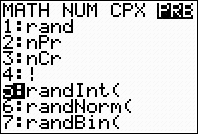Go to MATH → PRB Choose #5 randInt(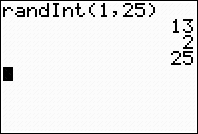From the home screen, enter the smallest value needed, followed by the largest value.  Hitting ENTER will generate the random integers one at a time. (Random values may repeat.) This example generates random integers from 1 to 25 which are good for Bingo games.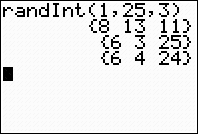Adding a third parameter indicates the number of random integers that will appear on the screen at one time.
 Generating Random Integers in Lists: (good for statistical studies)Go to MATH → PRB Choose #5 randInt(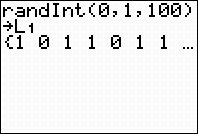From the home screen, enter the randInt followed by the smallest value in the desired range, the largest value, and the number of terms needed.  The results are stored (STO) into List 1. This example stores 100 random integers from 0 to 1 in L1 to simulate the toss of a coin.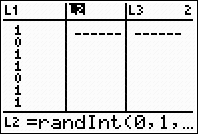OR, from the list screen, arrow up onto L2, and type randInt(0,1,100).  Hit ENTER. Be sure to enter the third parameter so the calculator will know "how many" numbers to place in the list.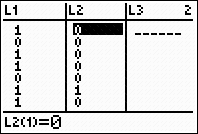Such lists can be used to simulate the toss of one (or more) fair coin(s).  The number of entries represents the number of tosses.  An even random number represents heads, while an odd number represents tails. If tossing one coin, use sum command to count the number of heads, where heads are 1, and tails are 0. 2nd STAT - MATH - #5 sum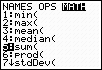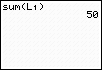Using the rand command: (not integers) The rand (MATH → PRB #1 rand) command will generate random values, not integers.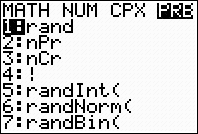MATH → PRB #1 rand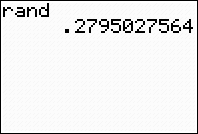The rand command will create a random number between 0 and 1.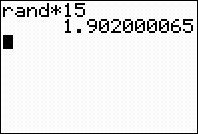To generate a random number between 0 and 15, enter rand*15.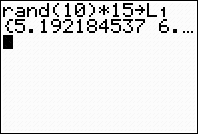This last entry shows how to generate a list of 10 random numbers between 0 and 15 and store them in List 1.
 Re-Seeding the Random Number Generator: Calculators (and computers) are not capable of creating "truly random" numbers.  They create what are called "pseudo-random" numbers, meaning they use a formula to create the values.  To engage this formula, the calculator uses a starting value, called a "seed", and then creates the random numbers based upon this seed.  If two calculators start with the same seed value, they will generate the same sequence of random values.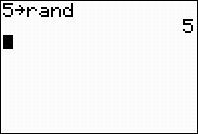If you wish, you can control the starting "seed" value. To seed the random generator, choose a seed value and store it into the rand command.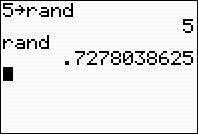Now, start generating your random values. If you feed two calculators the same seed value, they will each produce the same result when rand is entered.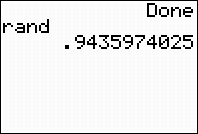After running a RESET (DEFAULTS) the calculator will return to using its default seed value.  Engaging rand will always produce the value seen above.  This same value will appear on all TI-84+ calculators after a reset. If you wish to ensure that each student in the class has a different set of random numbers, assign a different number to each student as their seed value.  You could also have the students enter their birth date as the random seed (04021990), assuming no two students have the same birth date.